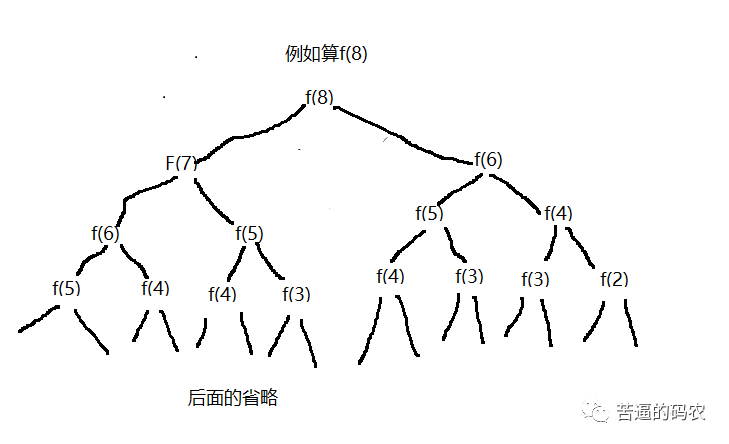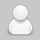2019
05-24

# 一些常用的算法技巧总结1. 巧用数组下标

``public void f(int arr[]) {        int[] temp = new int;        for (int i = 0; i < arr.length; i++) {            temp[arr[i]]++;        }        //顺序打印        for (int i = 0; i < 21; i++) {            for (int j = 0; j < temp[i]; j++) {                System.out.println(i);            }        }    }``

2. 巧用取余

`for (int i = 0; i < N; i++) {        if (pos < N) {         //没有越界         // 使用数组arr[pos]        else {          pos = 0;//置为0再使用数组           //使用arr[pos]         }        pos++;   }`

`for (int i = 0; i < N; i++) {   //使用数组arr[pos]   (我们假设刚开始的时候pos < N)   pos = (pos + 1) % N;}`

3. 巧用双指针

4. 巧用移位运算。

n / 2 等价于 n >> 1

n / 4 等价于 n >> 2

n / 8 等价于 n >> 3。

`if(n % 2 == 1){  dosomething();}`

`if(n & 1 == 1){  dosomething();)`

5. 设置哨兵位

6. 与递归有关的一些优化

（1）.对于可以递归的问题考虑状态保存

f(n) = f(n-1) + f(n - 2)。

`    public int f(int n) {        if (n <= 2) {            return n;        } else {            return f(n - 1) + f(n - 2);        }    }``//数组的大小根据具体情况来，由于int数组元素的的默认值是0    //因此我们不用初始化    int[] arr = new int;    public int f(int n) {        if (n <= 2) {            return n;        } else {            if (arr[n] != 0) {                return arr[n];//已经计算过，直接返回            } else {                arr[n] = f(n-1) + f(n-2);                return arr[n];            }        }    }`

(2).考虑自底向上

f(1) = 1;

f(2) = 2;

`public int f(int n) {        if(n <= 2)            return n;        int f1 = 1;        int f2 = 2;        int sum = 0;        for (int i = 3; i <= n; i++) {            sum = f1 + f2;            f1 = f2;            f2 = sum;        }        return sum;    }`

(1). 是否有状态重复计算的，可不可以使用备忘录法来优化。

(2). 是否可以采取递推的方法来自底向上做，减少一味递归的开销。

- End -